#### Chapter 10 Congruent Triangles R.D. Sharma Solutions for Class 9th MCQ's

Multiple Choice Questions

Q1. If ∆ABC ≅∆LKM, then side of ∆LKM equal to side AC of ∆ABC is
(a) LK
(b) KM
(c) LM
(d) None of these

Solution

Corresponding sides of congruent triangles are equal. The similarity is written accordingly. That means If ∆ABC ≅∆LKM, then corresponding equal sides will be
AB = LK
BC = KM
And, hence,
AC= LM
So, LM is correct answer, option  ( c) is correct.

Q2. If ∆ABC≅ ∆ACB, then ∆ABC is isosceles with
(a) AB=AC
(b) AB=BC
(c) AC=BC
(d) None of these

Solution

Corresponding sides of congruent triangles are equal. The similarity is written accordingly.
That means, If ∆ABC ≅∆ACB, then corresponding equal side of AB is AC
AB=AC
So, option (a) is correct.

Q3. If ∆ABC≅ ∆PQR and  ∆ABC is not congruent to ∆RPQ, which of the following is not true
(a) BC=PQ
(b) AC=PR
(c) AB=PQ
(d) QR=RC

Solution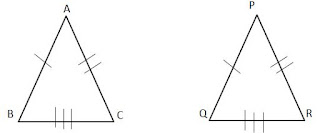Since,
∆ABC≅ ∆PQR
So,
Only corresponding parts are needed to be equal
So,
AB=PQ
AC=PR
BC=RQ
So, here, Option (c) AB=PQ is true
(b) AC=PR
(d) QR=BC are true.
Also, it is given that  ∆ ABC is not congruent to ∆RPQ.
So, BC≠PQ
So, Option (a) is correct answer.  BC=PQ  is not true.

Q4. In triangles ABC and PQR three equality relations between some parts are as follows.
AB=PQ, ∠B= ∠P and BC=PR
State which  congruence condition applies
(a) SAS
(b) ASA
(c) SSS
(d) RHS

Solution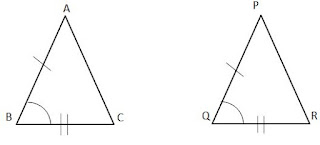Here are two triangles ABC and PQR
It is given
AB=PQ
∠B= ∠P
BC=PR
So, two sides are equal, and angle between these two equal sides are equal, therefore, both the triangles are concurrent by Side Angle Side (SAS) Criteria. Here, option (A) is correct.

Q5. In triangle ABC and PQR, if ∠A=∠R, ∠B=∠P and AB=RP. Then which of the following congruence condition applies:
(a)SAS
(b) ASA
(c) SSS
(d) RHS

SolutionThe above diagram is based on the conditions given
∠A=∠R, ∠B=∠P and AB=RP
So, Two corresponding angles of both triangles are equal, and side between these two triangles is also equal, therefore, both triangles are concurrent and condition is Angle side Angle (ASA).  Thus, option (b) is correct.

Q6. If ∆PQR≅∆EFD, then ED=
(a) PQ
(b) QR
(c) PR
(d) None of these

Solution

Since,
∆PQR≅∆EFD,
Corresponding sides will be equal.
PQ=EF
QR= FD
PR=ED
So, ED=PR as corresponding sides of concurrent triangles are equal
So, here, option (c) is true.

Q7. If ∆PQR≅∆EFD, then ∠E=
(a) ∠P
(b) ∠Q
(c) ∠R
(d) None of these

Solution

Since,
∆PQR≅∆EFD
Corresponding Angles are equal
∠P=∠E
Since, Angle E is corresponding part of angle P.
So, here, option (a) is correct

Q8.  In a ∆ ABC, If AB=AC and BC is produced to D such that ∠ACD=100° , then ∠A=
(a) 20°
(b) 40°
(c) 60°
(d) 80°

Solution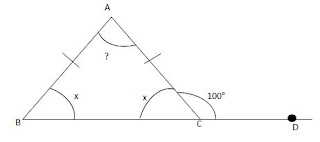The Above diagram is based on the question given
In ∆ABC, If AB=AC
Then, angles opposite to these sides will also be equal.
∠B=∠C = x (Let)
The Side BC of the triangle ABC is extended to D.
BCD is a straight line. So, ∠ACB+∠ACD=180°
So, x+100°=180° ( From the diagram) (It is given ACD=100° and we have assumed ∠ACB=100°.
x=180°-100°
⇒x=80°
That means ∠B=∠C = x= 80°
Since, Sum of all angles of triangle is 180°.
Therefore,
∠A+∠B+∠C=180°
⇒ ∠A+80°+80°=180°
⇒∠A+160°=180°
⇒∠A=180°-160°
⇒∠A=20°
So, ∠A=20° , hence, option (a) is correct.

Q9. In an Isosceles triangle, If the vertex angle is twice the sum of the base angles, then the vertex measure of the vertex angle of the triangle .
(a) 100°
(b) 120°
(c) 110°
(d) 130°

Solution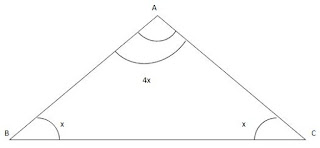Let the bases angles be x, then according to the question,  the vertex of angle according to the question is twice the sum of base angles
So, vertex angle is 2(x+x) = 2×2x = 4x
Sum of all angles will be 180°
So, x+x+4x=180°
⇒ 6x=180°
⇒ x=180°/6
⇒ x=30°
Vertex  Angle 4x = 4×30°=120°

Q10. D, E, F are the mid-points of the sides BC, CA and AB respectively of ∆ABC. Then ∆DEF is congruent  triangle.
(a) ABC
(b) AEF
(c) BFD, CDE
(d) AEF, BFD, CDE

SolutionAll, AEF, BFD, CDE are concurrent to each other.
Since the segment joining the mid points of any two sides of a triangle is half the third side and parallel to it.
DE = 1/2 AC ⇒ DE = AF = CF
EF = 1/2 AB  ⇒ EF = AD = BD
DF =   1/2 BC ⇒ DF = BE = CE
In △DEF and △CFE , we have
DE = CF [from (i)]
DF = CE [from (ii)]
EF = FE [common]
⇒ △DEF ≅ △CFE [by SSS criterion of congruence ]
Similarly, we have △DEF ≅  △BDE and △DEF ≅ △AFD
Thus, △DEF ≅  △CFE ≅ △BDE  ≅ △AFD
Hence, △ABC is divided into four congruent triangles
Q11.  Which of the following is not a criterion for congruence of triangles?
(a) SAS
(b) SSA
(c) ASA
(d) SSS

Solution

SAS, ASA and SSS are criterion for congruence of triangle. SSA is not a criterion. It is the criterion, but in special condition, that is the RHS criteria, SSA can be the condition. In general it is not. So, option (b) is the correct Answer.

Q12. In fig 10.135, the measure of ∠B’A’C’ is
(a) 50°
(b) 60°
(c) 70°
(d) 80°Solution

From the figure
AB=A’B’=6cm
BC=B’C’ =5cm
∠B=∠B’=60°
So, By SAS Criteria,
∆ABC≅∆A'B'C'
So,
∠A=∠A’
⇒3x=2x+20
⇒3x-2x=20
⇒x=20
We have to find
∠A’B’C’=2x+20
=2×20+20=60
So, ∠A’B’C’=60°
Hence, here, option (b) is correct.

Q13. If ABC and DEF are two triangles such that
∆ABC≅∆FDE and AB=5cm, ∠B=40° and ∠A=80°. Then which of the following is true?
DF=5cm, ∠F=60°
DE=5cm, ∠E=60°
DF=5cm, ∠E=60°
DE=5cm, ∠D=40°

SolutionThe above diagram, is according to question.
Since, Sum of all angles is 180°
∠A+∠B+∠C=180°
⇒80°+40°+∠C=180°
⇒120°+∠C=180°
⇒∠C=180°-120°=60°
Now, Since, ∆ABC≅∆FDE
Corresponding sides and angles will be equal
That means
DF = AB = 5cm
∠F=∠A=80°
∠E=∠C=60°
So, DF= 5cm, and ∠E=60°
That is option (c) here. So, option (c)  DF=5cm, ∠E=60° is  correct.

Q14. In figure, 10. 136, AB ⟂BE and FE ⟂BE.  If BC=DE and AB=EF, then ∆ABD is congruent to
(a) ∆EFC
(b) ∆ECF
(c) ∆ CEF
(d) ∆FECSolution

From the diagram
BC=DE
BC+CD=DE+CD
BD=EC
Also, it is given AB ⟂BE and FE ⟂BE
That makes
∠ABD=90°
∠FEC=90°
Now consider triangles ABD and FCE
AB=FE (given in question)
∠ABD=∠FEC=90°
BD=EC
So, by SAS criteria, ∆ABD≅∆FEC
So, here, ∆ABD is congruent with ∆FEC.
So, here Option (D) is correct.

Q15.  ABC is an Isosceles triangle Such that AB=AC and AD is median to base BC. Then ∠BAD=
(a) 55°
(b) 70°
(c) 35°
(d) 110°

SolutionFor Isosceles triangles, the median of unequal side, is perpendicular to the side.
So, AD is perpendicular to side AC
So,
In triangle ABD,
So, ∠BAD=55°, hence, here option (a) is correct.

Q16. In fig 10.138, if AE II DC, and AB=AC, the value of ∠ABD is
(a) 70°
(b)  110°
(c) 120°
(d) 130°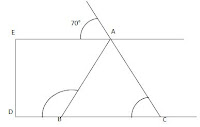SolutionSince, AE∥DC
We can say,
∠ACB=∠1=70° (corresponding Angle)
Since,
AB=AC
Triangle ABC is isosceles triangle.
So, angles opposite to equal side will be equal
∠ABC=∠ACB=70°
Since, CD is straight line, ∠ABC and ∠ABD are linear pair
Therefore,
∠ABC+∠ABD=180°
70°+∠ABD=180°
∠ABD=180°-70°=110°
So, ∠ABD=110° , hence, here option B is correct

Q 17. In fig 10.139, ABC is isosceles triangle whose sides AC is produced to E. Through C, CD is drawn parallel to BA. The value of xSolution

Since, opposite angles of equal sides of the isosceles triangle are equal,  therefore
∠ACB=∠ABC=52°
∠ACB+∠ABC+∠BAC=180°
⇒ 52°+52°+∠BAC=180°
⇒ 104°+∠BAC=180°
∠BAC=180°-104°
∠BAC=76°
Since, AB II CD
∠ACD=∠BAC=76°
Since, ∠ACD and ∠CDE are linear pair.
∠ACD+∠CDE=180°
76°+x°=180°  (from diagram, ∠CDE=x°)
⇒ x =180°-76°=104°
So, x=104, hence, here option (d) is correct.

Q18. In figure, 10.140, X is a point in the interior of the square ABCD. AXYZ is also a square. If DY=3cm, and AZ=2cm, then BY=
(a) 5cm
(b) 6cm
(c) 7cm
(d) 8cm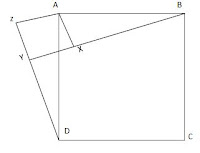Solution

Since, sides of square are equal
ZY=YX=XA=AZ=2cm
Consider ∆DAZ and ∆BAX
AD = BA (sides of Square ∆BCD)
AZ=AX (Sides of Square ∆XYZ)
∠DZA=∠BXA=90° (Angles of square ∆XYZ)
So, by RHS Criteria, ∆DAZ≅∆BAX
So, BX=DZ  (c.p.c.t)
Also,
From the diagram
BX=DY+YZ
⇒ BX=3cm+2cm = 5cm
⇒ BX=5cm

Q19. In fig 10.141, ABC is triangle in which ∠B=2∠C. D is a point on Side BC such that AD bisects ∠BAC and AB=CD. BE is bisector of ∠B. The measure of ∠BAC is
(a) 72°
(b) 73°
(c) 74°
(d) 95°SolutionGiven,
∠B=2∠C
⇒∠B/2 = ∠C
Also,
BE bisects ∠ABC
So, ∠ABC /2 = ∠ABE = ∠EBD
So, finally
∠ABE = ∠EBC = ∠C =∠ABC/2 = x (Let)
Or
∠ABC = 2x
Also,
∠BAD= ∠DAC = ∠BAC/2  =y (Let)
Or
∠A =2y

Considering ∆BEC
∠EBC = ∠ECB
So, BE= EC
Also, It is given AB=CD

So, considering ∆ABE and ∆DCE,
BE=EC
AB=CD
∠ABE = ∠DCE =x (from diagram)
So,
∆ABE≅ ∆DCE (SAS Criteria)

So,
∠EDC = ∠BAE = 2x  (c.p.c.t)
AE = DE (c.p.c.t)

In ∆AED
Since, AE = DE
∠EDA= ∠EAD = y (angles equal to opposite side)
From diagram
⇒ y+3y+x=180°
⇒ x+4y=180° --- (i)
Also, in triangle ABC
∠A+∠B+∠C = 180°
⇒ 2y+2x+x =180°
⇒ 2y+3x=180° --- (ii)
So, we have two equations
x+4y =180° (i)
2y+3x=180° (ii)
Multiplying eq (i) by 3 and subtracting (ii) from that
3x+12y= 3(180°)
⇒ -3x-2y=-180°
⇒ 10y=2×180°
⇒ y=(2×180°)/10 = 36°
We have to find angle ∠BAC=2y = 2×36°=72°
So, here, option (a) is correct.

Q20. In fig 10.142, If AC is bisector of ∠BAD, Such that AB=3cm and AC=5cm, then CD=
(a) 2cm
(b) 3cm
(c) 4cm
(d) 5cmSolution

Since,
Also,
So, Considering ∆ ABC and ∆ADC
AC (common Side)
AB = AD = 3cm (c.p.c.t)